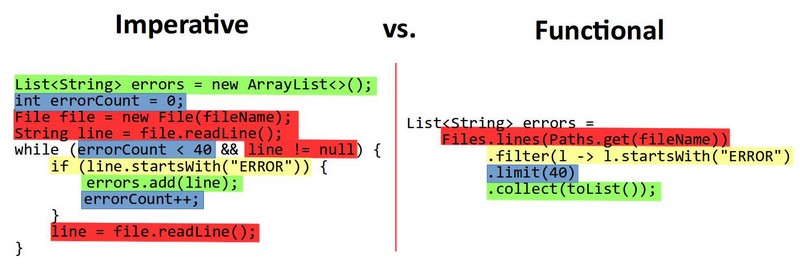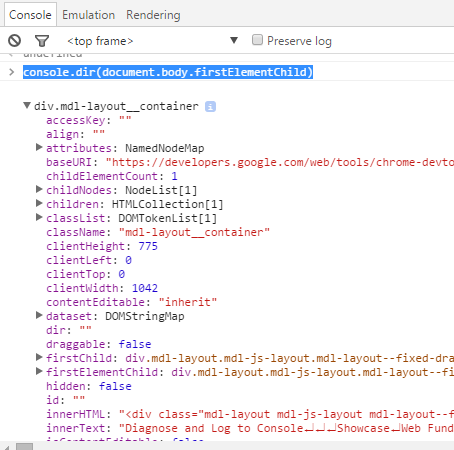# Javascript - Array

array in Javascript can contain any type of data. Different types of values can be stored within the same array

Because arrays are special objects (as typeof implies), they can also have properties, including the automatically updated length property.

## Property

### Key

An array is a Object Derived Type (ie an object) , therefore the key of an array are the name of a property where the names are automatically set to a automatic increasing sequence of number if not set.

Proof:

• creating a minimal array
````let a = ["a","b","c"];`
```
• The typeof the array variable
````console.log("The type of an array is "+typeof(a));`
```
• Adding a property to the object array
``````a["foo"]="d";
console.log("The value of a are: ")
console.log( a)
console.log("But the length is (not 4) but "+a.length);```
```

If you want a specific associative array implementation (ie a map), see the map data type article

## Management

### Initialization

#### Manually

• Empty Array
````var anEmptyArrays = []`
```
• Array with number
````var numberArrays = [1,2,3,4,5]`
```
````console.log(numberArrays)`
```
• Array with two rows
``````var arrayWithTwoRows = [[1,2,3], [2,3,4]]
console.log(arrayWithTwoRows)```
```

#### Generation

``````let integerArray = Array.from(Array(10), (element, index) => index+2);
console.log(integerArray);```
```

### Length

``````var numbers = [1,2,3,4,5]
console.log(numbers.length)```
```

### Type / is Array

The type of an array is an object. Luckily, to recognize a variable hosting an array, you can use the Array.isArray function.

``````var numbers = [1,2,3,4,5];

console.log(typeof numbers);
console.log(Array.isArray(numbers));```
```

It seems that the below expression should be true but it's false.

````console.log(Array.isArray({__proto__: []})); `
```

### Indexing

• Return the ith element. First element is on 0.
``````var arrayName = [1,2,3];
for (var i = 0; i < arrayName.length; i++) {
console.log(arrayName[i]);
}```
```

### Merge

``````let array1 = [0,2];
let array2 = [4,2];
// delete the value of the second array found in the first
let array2WithoutArray1Values = array2.filter(element => array1.indexOf(element) < 0)
// merge by position
let array3 = array1.concat(array2WithoutArray1Values);
// result
console.log(array3)```
```

### Concatenate

• With the concat method
``````var arr1 = [0, 2, 3];
var arr2 = [3, 4, 5];
arr1 = arr1.concat(arr2 );
console.log(arr1);```
```
``````var arr1 = [0, 2, 3];
var arr2 = [3, 4, 5];
arr1 = [...arr1, ...arr2];
console.log(arr1);```
```

### Insert

#### Splice

````arr.splice(index, deleting, item);`
```

will:

• insert item
• into arr
• at the specified index
• deleting items first
``````myArray = ["Nico","Rixt","Melissa"]
console.log(myArray);
console.log(myArray);```
```

``````var parts = ['shoulders', 'knees'];
var lyrics = ['head', ...parts, 'and', 'toes'];
console.log(lyrics);```
```

#### Push

Push inserts elements into an array one by one. In ES5 it's solved with .apply(): an unfriendly and verbose approach

``````// Syntax
array.push(item1, ..., itemN) ```
```

Example: push into an existing array, without creating a new instance. with the ES5 apply

``````var parts = ['head', 'shoulders', 'knees'];
var lyrics = ['and', 'toes'];
Array.prototype.push.apply(parts, lyrics);
console.log(parts); ```
```

### Remove

• Splice. Mutable operation.
``````const index = array.indexOf(element);

if (index !== -1) {
array.splice(index, 1);
}```
```
• Map. Immutable Operation (ie create a new array)
````newArray = array.filter(e => e !== element);`
```

The set contains a delete function

• All (keeping the reference)
````myArray.length = 0;`
```

### Copy

``````var arr = [1, 2, 3];
var arr2 = [...arr]; // like arr.slice()
arr2.push(4);

console.log("arr2 becomes "+arr2);
console.log("arr remains unaffected "+arr);```
```

arr.slice()

### Loop

#### For Of

``````var colors = [ "blue", "green" ];
console.log("color values are:");
for (const color of colors) {
console.log("  "+color);
}```
```

More for iteration, see the following page: Javascript - For Statement

#### For i

``````var colors = [ "blue", "green" ];
for (var i = 0; i < colors.length; i++) {
console.log(i+" "+colors[i]);
}```
```

More for iteration, see the following page: Javascript - For Statement

### Equality

Both == and === object equality operator will simply check whether the references match, not anything about the underlying values.

``````var a = [1,2,3];
var b = [1,2,3];
var c = "1,2,3";
console.log( a == c ); // true
console.log( b == c ); // true
console.log( a == b ); // false```
```

### Contains

Example;

• The array
````var a = [1,2,3]; `
```
• includes() method determines whether an array includes a certain value
``````console.log("2 is included in the array: "+ a.includes(2) );
console.log("4 is included in the array: "+ a.includes(4) ); ```
```
• the indexOf function may be also used with a predicate (indexOf return -1 if the element is not present)
``````console.log("2 is in the array: "+ (a.indexOf(2) !== -1) );
console.log("4 is in the array: "+ ( a.indexOf(4) !== -1) ); ```
```

### To String

#### Join

``````let a = ["a","b","c"];
console.log(a.join("/"));```
```

Discover MoreD3 - Operator

Operators act on selections, modifying content. Operator values are specified either as constants or functions; the latter (the functions) are evaluated for each element. (The functions are evaluated...
DOM - NodeList (Collection)

A nodelist is the result of a selection that returns more than one node (Generally an element) Nodelist: HTMLAllCollection, HTMLFormControlsCollection, HTMLOptionsCollection, interfaces are...Functional Programming - Map

A page the map functional programming function The map method creates a new collection with the results of calling a provided function on every element in the collection. The map operation produces...
How to sort an array of objects in Javascript?

This example shows you how you can sort an array of objects with the sort functionJavascript - (Console) Logging

logging in javascript are functions of the console Console logging permits inspecting a Web application (page). window Level Shows All Shows all console output Errors Only show output from...
Javascript - Boolean (Truthy|Falsy)

in Javascript. JavaScript defines a list of specific values that are considered “falsy” because when coerced to a boolean, they become false. Any other value not on the “falsy” list is automatically...
Javascript - For Statement

The for iterator statement array iteration The colors Reference/Statements/for...in. array The loop iterate over all enumerable properties of the object itself and those the object inherits...
Javascript - Functional Programming

in javascript. array operationsDoc To get the cookies All function returns an array. A few returns an element...
Javascript - Map function (Functional programming)

The functional programming function map in a Javascript context is only an array method as all functional programming javascript method. map With an Arrow function as argument Letters that we...
Javascript - Object Equality

This page is talking Equality for an object in the sense, not a primitive. For a comparison between object, both == and === object equality operator will simply check whether the references match....